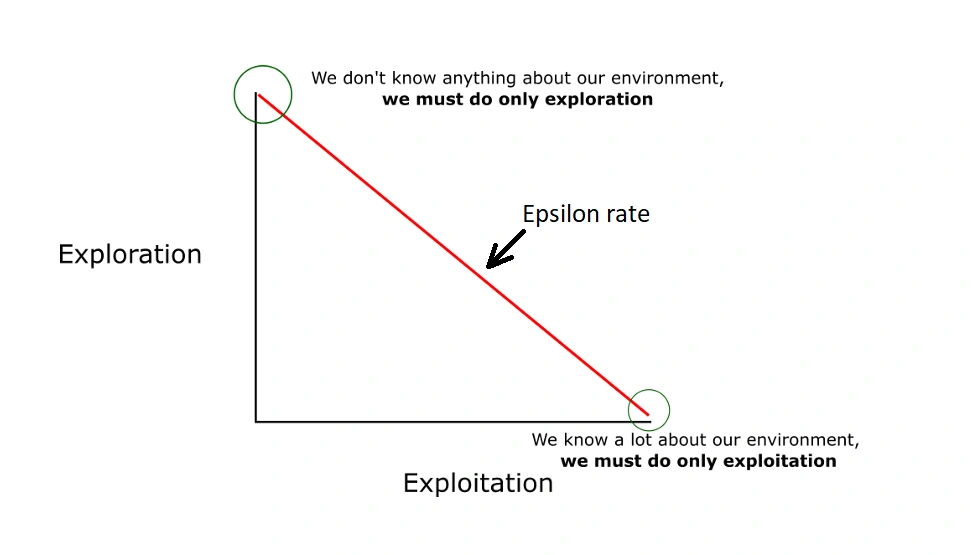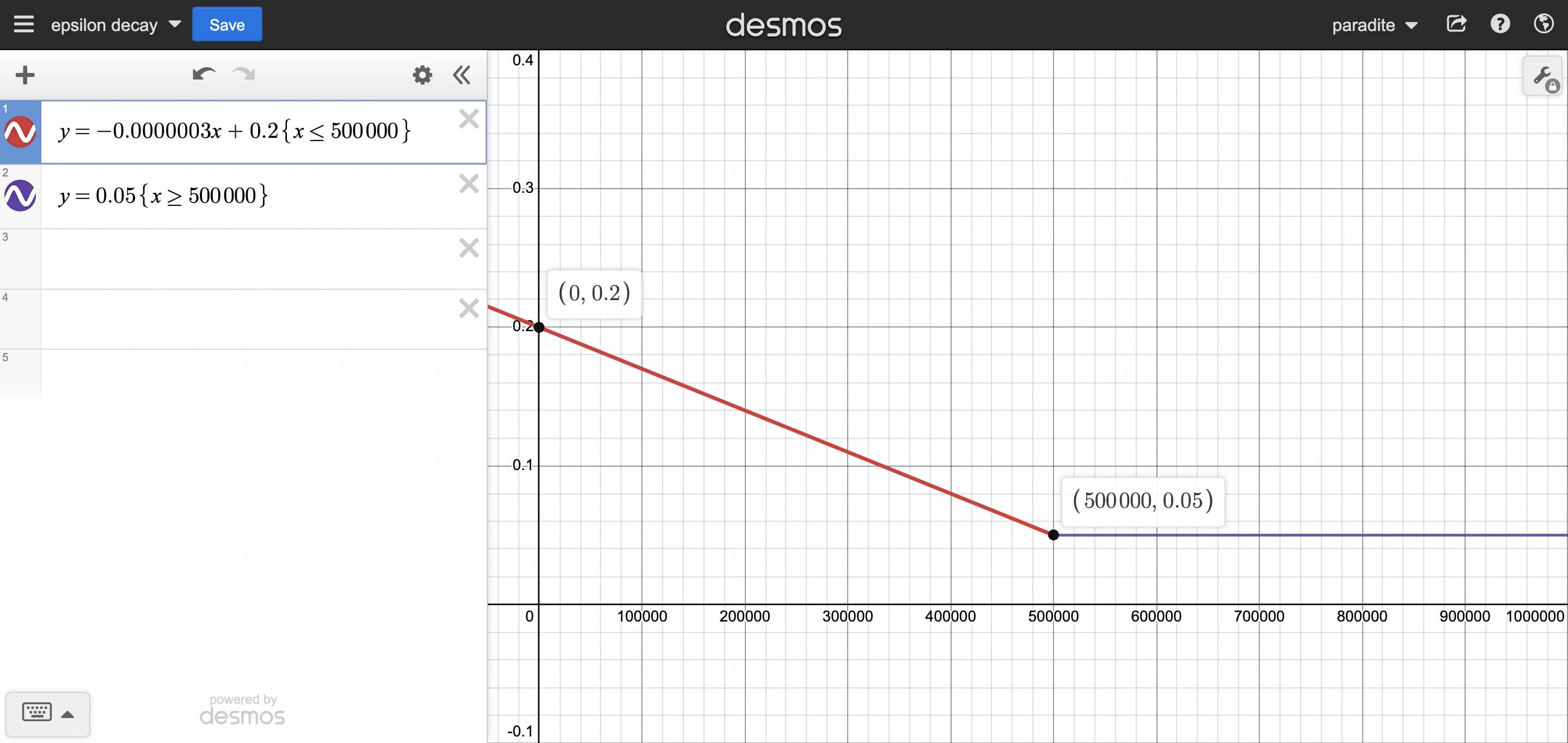Epsilon decay refers to decreasing the epsilon value over time.

With epsilon delay, epsilon gradually decreases from initial epsilon to final epsilon over a fixed number of frames (steps), called “epsilon decay frames”.

Epsilon decay is a method to balance “exploitation and exploration”. At the start, high epsilon is used to explore the environment. At the end, low epsilon is used to exploit the environment.The model will continue to learn with final epsilon after completing the “epsilon decay frames”.

### Example

0.2 initial epsilon, 0.05 final epsilon, 500k frames means epsilon will decay from 0.2 to 0.05 in 500k frames, and continue to train with epsilon at 0.05.